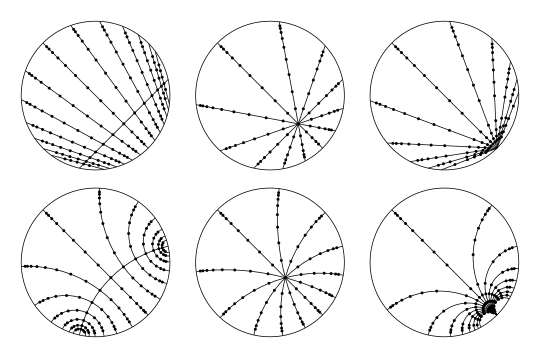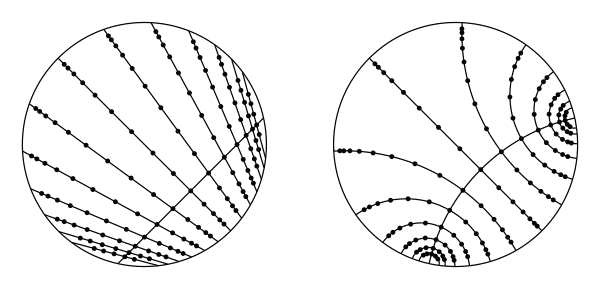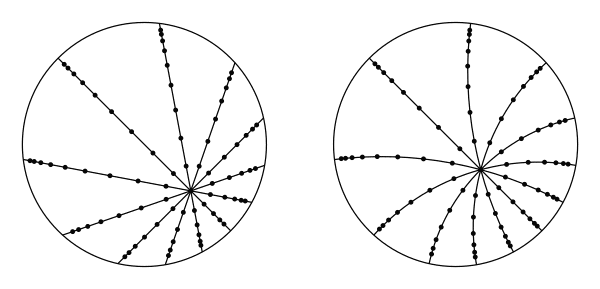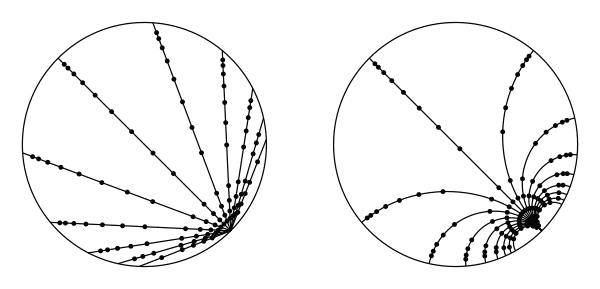# Hyperbolic plane isometry

An isometry of the hyperbolic plane is a mapping of the hyperbolic plane to itself that preserves the underlying hyperbolic geometry (e.g. distances and angles).

The isometries of the hyperbolic plane form a group under composition.

An isometry of the hyperbolic plane can be either orientation-preserving or orientation-reversing. We discuss orientation-preserving isometries first after introducing some preliminaries.This figure shows an animation of the three types of orientation-preserving isometries of the hyperbolic plane (from left to right): hyperbolic, elliptic, and parabolic. The first row uses the Klein or projective model, and the second row the Poincaré disk model.

## Preliminaries

Throughout this article we use $$H$$ to denote the hyperbolic plane and $$\overline{H}$$ to denote the hyperbolic plane together with its boundary at infinity. We also assume $$H$$ has an orientation. This lets us talk about things like "clockwise" and "left" or "right" in $$H$$.

We also sometimes identify $$H$$ with a model of the hyperbolic plane like the Klein model, the Poincaré disk model, or the upper half-space model, which can all be viewed as subsets of the $$xy$$- or complex plane. We assume these identifications always preserve orientation. This ensures that things like "clockwise" and "left" or "right" in $$H$$ match what they would be if inherited from the complex or $$xy$$-plane.

## Orientation-preserving isometries

The orientation-preserving isometries of the hyperbolic plane form a subgroup of the full group of isometries. This subgroup equals $$PSL(2, \mathbf{R})$$ (e.g. by the latter group's action on the upper half-plane model of the hyperbolic plane). We will sometimes discuss isometries from the perspective of $$PSL(2, \mathbf{R})$$, so for the purposes of this section, we'll assume we have an identification of the group of orientation-preserving isometries with $$PSL(2, \mathbf{R})$$.

Thus, given an orientation-preserving isometry of the plane, we can view it as an element of $$PSL(2, \mathbf{R})$$. We can then choose a matrix $$M$$ in $$SL(2, \mathbf{R})$$ that represents that element. This matrix will be unique up to multiplication by $$-1$$. We will call such an $$M$$ a matrix that represents the isometry.

There are three types of orientation-preserving isometries of the hyperbolic plane: hyperbolic, elliptic, and parabolic. (This terminology can be confusing at first because "hyperbolic isometry" can be used both to mean any isometry of the hyperbolic plane, as well as only those isometries that have hyperbolic type. In general, readers will have to pay attention to the context to know which meaning is being used.)

There is also a fourth type of isometry sometimes left out because it is trivial: the identity isometry. The identity isometry can also be thought of as a degenerate form of the other three types (e.g. an elliptic isometry with an angle of rotation of zero).

Whether an isometry is hyperbolic, elliptic, parabolic, or the identity is a conjugacy invariant in the full group of isometries of the hyperbolic plane.

One geometric way to tell the type of an isometry is by the nature and number of the isometry's fixed points in $$\overline{H}$$. For example, the identity isometry is the unique isometry that fixes all points in $$H$$ (as well as in $$\overline{H}$$). A more algebraic way is to choose a matrix $$M$$ in $$SL(2, \mathbf{R})$$ representing the element and then examine the trace or eigenvalues of $$M$$. In addition to the qualitative information of the type, the trace and eigenvalues also provide quantitative information about the isometry. We will discuss all of this in more detail below.

### Hyperbolic isometry

A hyperbolic isometry is an orientation-preserving isometry with exactly two fixed points in $$\overline{H}$$. These two points will necessarily be in the boundary at infinity.This figure shows an animation of a family of hyperbolic isometries with increasing translation length. Both sides show the same isometries, with the left using the Klein model, and the right using the Poincaré disk model. Along the geodesics, the dots are spaced a distance 0.25 apart in the Klein model, and 0.5 apart in the Poincare disk model.

A hyperbolic isometry is sometimes called a "translation." A hyperbolic isometry fixes (as a set) the geodesic that connects its two fixed points. This geodesic is sometimes called its "axis." The isometry translates all points in this geodesic by a common distance. This distance is called the isometry's translation length.

A hyperbolic isometry is determined by (1) its two fixed points, or equivalently the geodesic it fixes, (2) a direction, or orientation of the geodesic it fixes, and (3) its translation length. The translation length is invariant under conjugation in the full group of isometries of the plane, and all hyperbolic isometries with the same translation length are conjugate to one another via an orientation-preserving isometry.

Let $$T$$ be any hyperbolic isometry, and let $$k>0$$ be its translation length. Viewing the hyperbolic plane using the upper half-plane model, conjugate $$T$$ to a hyperbolic isometry that preserves the $$y$$-axis and translates it in the positive direction. In the upper half-plane, such an isometry takes the form of scalar multiplication of the complex plane by $$e^k$$. Let $$M$$ be any matrix in $$SL(2, \mathbf{R})$$ representing this isometry. The matrix is determined up to sign, so $$M=\pm\begin{pmatrix} e^{k/2} & 0 \\ 0 & e^{-k/2} \end{pmatrix}$$.

Observe that the trace equals $$\operatorname{tr}(M)=\pm(e^{k/2} + e^{-k/2})$$. Since $$k\ne 0$$, the trace satisfies $$|\operatorname{tr}(M)|>2$$. The translation length can be recovered from the trace by $$k=2\cosh^{-1}(|\operatorname{tr}(M)|/2)$$. This follows by applying the definition of hyperbolic cosine $$\cosh x=(e^{x} + e^{-x})/2$$.

Similarly, the translation length can be recovered directly from the eigenvalues (or an eigenvalue) of $$M$$. If we let $$\lambda=\lambda_1=e^{\pm k/2}$$ and $$\lambda_2=e^{\mp k/2}$$ be the two eigenvalues (being blind to which is which), then we have $$|\log(\lambda_1/\lambda_2)|=|\log(\lambda^2)|=k$$, or $$k=2|\log \lambda|$$.

Since the trace and eigenvalues are invariant under matrix conjugation, the formulas above hold for any matrix $$M$$ in $$SL(2, \mathbf{R})$$ representing the hyperbolic isometry $$T$$ (and not just after conjugating $$T$$ as we did above). In particular, $$|\operatorname{tr}(M)|>2$$, and, letting $$\lambda$$ be either of the eigenvalues, $$\lambda$$ is real and $$\lambda\ne \pm 1$$. In addition, for the translation length $$k$$, we have $$k=2|\log \lambda|=2\cosh^{-1}(|\operatorname{tr}(M)|/2)$$.

### Elliptic isometry

An elliptic isometry is an isometry with exactly one fixed point in $$\overline{H}$$, and with the fixed point in $$H$$ rather than in the boundary.This figure shows an animation of a family of elliptic isometries with a continually increasing angle of rotation (in the clockwise direction). See the caption for the hyperbolic isometry animation for more info about this figure.

An elliptic isometry is sometimes called a "rotation." An elliptic isometry is determined by (1) its fixed point, and (2) its angle of rotation. An isometry's angle of rotation is invariant under conjugation by orientation-preserving isometries and is invariant up to sign under conjugation in the full group of isometries. All elliptic isometries with the same angle of rotation are conjugate to one another via an orientation-preserving isometry.

Let $$E$$ be any elliptic isometry, and let $$\theta$$ be its angle of rotation, choosing $$-\pi<\theta\leq\pi$$ and with counter-clockwise being the positive direction. Since $$E$$ is not an isometry, we have $$\theta\ne 0$$. Viewing the hyperbolic plane using the upper half-plane model, conjugate $$E$$ via an orientation-preserving isometry to an elliptic isometry whose fixed point is $$i$$ (i.e. the "center" of the upper half-plane). Then the angle of rotation is the same. Let $$M$$ be any matrix in $$SL(2, \mathbf{R})$$ representing this new isometry. The matrix is determined up to sign and takes the form $$M=\pm\begin{pmatrix} \cos\frac{\theta}{2} & \sin\frac{\theta}{2} \\ -\sin \frac{\theta}{2} & \cos \frac{\theta}{2} \end{pmatrix}$$.

Observe that the trace equals $$\operatorname{tr}(M)=\pm2\cos \frac{\theta}{2}$$. Since $$\theta$$ is not a multiple of $$2\pi$$, the trace satisfies $$|\operatorname{tr}(M)|<2$$. The angle of rotation can be recovered from the trace, but only up to sign (aka direction) since cosine is an even function. Specifically, we have $$\theta=\pm 2\cos^{-1}(|\operatorname{tr}(M)|/2)$$.

Alternatively, the angle of rotation can be recovered from the eigenvalues of $$M$$, again up to sign, as follows. The eigenvalues of $$M$$ can be computed to be the complex numbers $$e^{i\theta/2}$$ and $$e^{-i\theta/2}$$ on the unit circle. If we let $$\lambda=\lambda_1=e^{\pm i\theta/2}$$ and $$\lambda_2=e^{\mp i\theta/2}$$ be the eigenvalues (again being blind to which is which), we have $$|\log(\lambda_1/\lambda_2)|=|\log(\lambda^2)|=|\theta|$$, or $$\theta=\pm 2|\log \lambda|$$.

Since the trace and eigenvalues are invariant under matrix conjugation, the formulas above hold for any matrix $$M$$ in $$SL(2, \mathbf{R})$$ representing the elliptic isometry $$E$$ (so not just after conjugating $$E$$). In particular, $$|\operatorname{tr}(M)|<2$$, and, letting $$\lambda$$ be either of the eigenvalues, $$\lambda$$ is not real and lies on the complex unit circle. In addition, for the angle of rotation $$\theta$$, we have $$\theta=\pm 2|\log \lambda|=\pm 2\cos^{-1}(|\operatorname{tr}(M)|/2)$$. These parallel the corresponding expressions in the hyperbolic isometry case.

### Parabolic isometry

A parabolic isometry is an isometry with exactly one fixed point in $$\overline{H}$$, and with the fixed point in the boundary rather than in $$H$$.This figure shows an animation of a family of parabolic isometries with a continually increasing distance by which points are moved. See the caption for the hyperbolic isometry animation for more info about this figure.

Choose an arbitrary point $$p$$ in $$H$$ (so not on the boundary). A parabolic isometry is then determined by (1) its fixed point, (2) the distance it moves $$p$$, and (3) the direction it moves $$p$$ (e.g. left or right when facing $$H$$ from the fixed point). The direction of a parabolic isometry is invariant under conjugation by orientation-preserving isometries but not by orientation-reversing isometries. In the full group of isometries of the plane, all parabolic isometries are conjugate to one another (i.e. so there is just one conjugacy class of parabolic elements). In the group of orientation-preserving isometries, only all parabolic isometries of the same direction are conjugate to one another (so there are two conjugacy classes in this case).

The fact that all parabolic isometries with the same direction are conjugate to one another explains why we had to fix a point $$p$$ to associate a translation distance with the isometry. The distance isn't intrinsic to the isometry itself.

Let $$P$$ be any parabolic isometry, and let $$p$$ be its fixed point on the boundary. Then $$P$$ moves all points in the boundary in the same direction along the boundary (except for $$p$$, which is fixed). For the purposes of this section, we say $$P$$ is "positive" if $$P$$ moves these points in the counter-clockwise direction, and "negative" otherwise.

Viewing the hyperbolic plane using the upper half-plane model, conjugate $$P$$ using an orientation-preserving isometry to a parabolic isometry whose fixed point is the point at infinity in the complex plane. Let $$M$$ be any matrix in $$SL(2, \mathbf{R})$$ representing this new isometry. The matrix is determined up to sign and takes the form $$M=\pm \begin{pmatrix} 1 & \pm 1 \\ 0 & 1 \end{pmatrix}$$. The sign of the $$1$$ in the upper-right corner is positive if $$P$$ is positive, and negative if $$P$$ is negative. In the upper half-plane model, $$M$$ acts by mapping $$(x, y) \mapsto (x+1, y)$$ if $$P$$ is positive and $$(x, y) \mapsto (x-1, y)$$ if $$P$$ is negative. In other words, $$M$$ shifts the upper half-plane to the right or left by $$1$$, depending on whether $$P$$ is positive or negative, respectively.

Finally, observe that the trace equals $$\operatorname{tr}(M)=\pm2$$, which is true also for the identity isometry. Since the trace is invariant under matrix conjugation, this holds for any matrix $$M$$ in $$SL(2, \mathbf{R})$$ representing a parabolic isometry $$P$$. Thus, it is a distinguishing property of parabolic isometries (and of the identity isometry) that $$|\operatorname{tr}(M)|=2$$.

### Orientation-preserving summary

This subsection summarizes some of the key information about the types of orientation-preserving isometries of the hyperbolic plane. Let $$O$$ be any orientation-preserving isometry of $$H$$. Let $$M$$ be a matrix in $$SL(2, \mathbf{R})$$ representing $$O$$, and let $$\lambda$$ be an eigenvalue of $$M$$.

• $$O$$ is elliptic if any of the following equivalent conditions are satisfied:
• $$O$$ has a single fixed point in $$\overline{H}$$, and that point is not on the boundary of $$H$$.
• $$|\operatorname{tr}(M)|<2$$.
• $$\lambda$$ is not real (in which case $$\lambda$$ lies on the complex unit circle).
• $$O$$ is parabolic if any of the following equivalent conditions are satisfied:
• $$O$$ has a single fixed point in $$\overline{H}$$, and that point is on the boundary of $$H$$.
• $$O$$ is not the identity isometry, and $$|\operatorname{tr}(M)|=2$$.
• $$O$$ is not the identity isometry, and $$\lambda=\pm 1$$.
• $$O$$ is hyperbolic if any of the following equivalent conditions are satisfied:
• $$O$$ has two fixed points in $$\overline{H}$$ (in which case the points lie on the boundary).
• $$|\operatorname{tr}(M)|>2$$.
• $$\lambda$$ is real but $$\lambda\ne\pm 1$$.

## Orientation-reversing isometries

The classification of orientation-reversing isometries is much simpler than for the orientation-preserving case. For orientation-reversing isometries, there are only two types (or one, depending on how you count them): reflections and glide reflections.

### Reflection

A reflection is an isometry that results from reflecting across a geodesic.

For example, consider the geodesic in the upper half-plane model defined by the $$y$$-axis. The reflection corresponding to this geodesic is defined by the mapping $$(x, y)\mapsto(-x, y)$$.

The set of fixed points in $$\overline{H}$$ for a reflection is exactly the set of points making up the geodesic, including the geodesic's endpoints at infinity. A reflection is uniquely determined by its geodesic, and every reflection is conjugate to every other one by an orientation-preserving isometry (so there is just one conjugacy class).

### Glide reflection

A glide reflection is an isometry that results from composing a reflection with a non-trivial translation (aka hyperbolic isometry) along the geodesic corresponding to the reflection. (If we were to allow translations of length zero, then a reflection would be a type of glide reflection. This is the sense in which one could classify orientation-reversing isometries with just one type.)

Just like with hyperbolic isometries, a glide reflection has exactly two fixed points, namely the endpoints at infinity of the geodesic corresponding to the reflection and translation.

Also like hyperbolic isometries, a glide reflection is determined by (1) its two fixed points, or equivalently the geodesic it fixes, (2) a direction, or orientation of the geodesic it fixes, and (3) a positive translation length. All glide reflections with the same translation length are conjugate to one another.